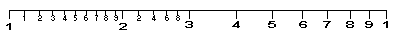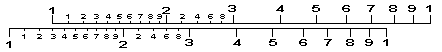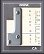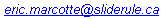# Introduction to the Slide RuleThe words "slide rule" may conjure up images of overwhelming complexity and technical detail for you, but the underlying concept is actually quite straightforward. At its heart, the slide rule is nothing more than a simple and elegant solution for solving basic mathematical calculations. What I'll attempt to do on this page is to explain where the slide rule came from, and how it works. Don't worry about all that intimidating detail you see on the face of a slide rule ... you'll quickly see that it is actually quite simple to understand and use (or, in the words of Douglas Adams, DON'T PANIC!). Although the slide rule goes by many names (sliderule, slide ruler, slipstick, etc.) and comes in many forms (linear, circular, cylindrical, etc.), the background presented below applies equally well to all makes and models.

If you want to skip the theory, and proceed directly to how to use a slide rule, see my How to Use a Slide Rule page for some downloadable manuals. You might also want to check out the various types of rules and the meanings of common scales listed on my Slide Rule Scales page. Incidentally, all the sources for the historical background presented here can be found in the Further Reading section at the bottom of this page.

### To Begin ....

Probably the best way to illustrate what a slide rule can do is to start by what it typically won't do; namely, addition or subtraction. This might surprise you at first, but the reasons why this is so will help you to understand the basic structure and use of a slide rule. Actually, addition and subtraction are integral to slide rule function, just not in the way you are probably accustomed to. To illustrate this principle, look at the following column consisting of three numbers:

324
520
112

If I were to hand you pencil and paper and ask you to add all those numbers together (that is, 324 + 520 + 112), it would probably take you only a few seconds to tell me the correct answer of 956. But what if I ask you instead to multiply all of them together (that is, 324 X 520 X 112)? I could probably go for a coffee, and by the time I got back you would still be checking your answer! But with any standard 10 inch slide rule, you could tell me the answer was a little less than 18.9 million in about the same amount of time as the addition problem would have taken you by hand. The exact answer is 18,869,760, but you wouldn't be able to tell that with a slide rule for reasons that will become clearer a bit later. The point here is that quickly knowing that the answer is just under 18.9 million is often good enough for most applications. The slide rule accomplishes this little trick of mathematics by using logarithms (don't worry, it's actually quite simple).

### Basis of a Slide RuleLogarithms (or logs, as they are known in short) were invented by the Scottish mathematician and theologian John Napier and first published in 1614. He was looking for a way of quickly solving multiplication and division problems (like the one above) using the much faster methods of addition and subtraction. Napier's way of doing this was to invent a group of "artificial" numbers as a direct substitute for real ones. He called this numbering system logarithms (which is Greek for "ratio-number", apparently). Logarithms are consistent, related values which substitute for real numbers. Incidentally, it wasn't until a few years later, in 1617, that a fellow mathematician named Henry Briggs adapted Napier's original "natural" logs to the more commonly used and convenient base 10 format. Here is how logs work:

To start, the log of the real number 1 is 0. The log of the real number 10 is 1. The log of the real number 100 is 2. The log of the number 1,000 is 3. And so on, and so on. This might already sound familiar to you - it is just like the Richter scale for earthquakes, or the pH scale for acids and bases. Just as an earthquake measuring 5 on the Richter scale is actually 10 times worse than one measuring 4, the log value 5 is actually 10 times larger than the log value 4. But what happens to numbers falling in-between factors of 10? Well, any number between 1 and 10 is going to have a log value between 0 and 1. Similarly, any number between 10 and 100 is going to have a log value between 1 and 2. And so on, and so on. More importantly, though, every number is faithfully related to every other number in the same fashion within those scales. For example, the log of the real number 3 is 0.4771. The log of the real number 30 is 1.4771. The log of the real number 300 is 2.4771, and so on, and so on. As you can see, all these numbers fall in exactly the same place between the base 10 units.

To see how these are useful for multiplication, consider what happens if you want to multiply 10 X 1,000, as a simple example. The secret here is that you could just add their logs together and then take the anti-log of the result. So, if the log of 10 is 1, and the log of 1,000 is 3, then the sum of 1 + 3 is 4. Notice that 4 is also the log of 10,000, which just happens to be the correct answer! The same rule applies for division. If you wanted to divide 1,000 by 10, you would simply subtract the log of 10 (that is, 1) from log of 1,000 (3) which would get you 3 - 1 = 2, which is the log of correct answer, namely 100. As you may have guessed by now, the same rule applies for any number in-between those powers of ten. So, to go back to the example at the top of the page, we can multiply those three numbers together by simply adding their logs. For this, you can either use a pocket calculator, or look up the values in standard log tables, as follows:

log of 324 = 2.5105
log of 520 = 2.7160
log of 112 = 2.0492

Sum of logs = 7.2757

Now, the first thing you can tell from the answer is that it is going to be in the tens of millions (just as the log value 2 refers to the real number 100, a log value of 7 would refer to the real number 10 million). This "characteristic" part of a log (the number to the left of the decimal) is very useful in determining the magnitude of your answer. The part on the right of the decimal point (known as the mantissa) can be looked up in any log table, and you will find that the final answer corresponds to a number that is between 18.8 and 18.9 million, and closer to the later. Due to number of significant figures involved, you really can't make a statement to any greater precision (the related concepts of significant figures, precision, and accuracy are explained at the bottom of this page). So, although you could have done the multiplication the hard way, I hope you can see how useful having a set of log tables would be instead.

### The Development of the First Slide Rule

The next step in the evolution of the slide rule was really an exercise in visualization. It is hard to get a feel for what the log of any given number will be, exactly, without looking it up in the standard reference tables. But what if instead you drew them down to give you a rough idea of where things lay? This is exactly what the English astronomer Edmund Gunter came up with in 1620. He drew a 2 foot long line with the whole numbers spaced at intervals proportionate to their respective log values (see image below, taken from HP's slide rule site). You could now take a bunch of dividers (or calipers, if you like) and measure off the differences between numbers, as an aid in doing calculations without having to refer to tables. Notice, by the way, how all the numbers seem "squished" at the right end of the log scale, and "spread out" and the lower end. This mechanical illustration of the log scale is really key to the development of the slide rule.We are now just a short jump away from the first real slide rule, developed by the Reverend William Oughtred a short time later. He placed two Gunter's scales directly opposite each other, and demonstrated that you could do calculations by simply sliding them back and forth (see the second image below). As such, Oughtred is generally considered to be the inventor of the slide rule, although there has been considerable debate as to when exactly he came up with his invention (it was probably within a few years of Gunter's original line). The relative role of a number of people in the development of the original slide rule concept (including Oughtred, Edmund Wingate, and several of Oughtred's and Gunter's students, among others) is an area of intense historical interest for followers of such things.Notice here is that it is not necessary to go on forever with log scales in order to calculate big numbers. Like Gunter's line, you just need to go from 1 to 10, with a large number of subdivisions underneath, and you can determine the final answer just like we did in the earlier example using log tables. By placing another log scale (ranging from 1 to 10) within 1 to 2 on the main scale, we can now look at all the log numbers between 10 and 20 this way. Notice that this sub-scale still has the same stretched-out appearance as the main scale. The more scales you can fit in the subdivisions, the more decimal places you can talk about it your final answer (more on that in a moment).

One problem with the slide rule method is that keeping track of where the decimal point should be is a bit tricky. Simply put, it is hard to keep track of the characteristic of the logs with slide rules. To use our earlier example, are you sure the correct answer is 18.9 million, or is it maybe 1.89 million? Or for that matter, maybe 0.189? As you can see, knowing where to put the all-important decimal point is key. I won't get into the details here, but there is an excellent discussion of the various strategies developed for determining decimal points with a slide rule at Ron Manley's Slide Rule site. In case you are curious, I prefer the approximation method in simple cases, and the scientific notation method for more advanced jobs. Incidentally, this decimal point problem illustrates why it wouldn't be very convenient to do basic addition or subtraction with a slide rule using regular linear scales. Although you certainly could design a rule this way, what we would be the point when every calculation would require you to keep track of the decimal point? It is far easier to just use paper and pencil the old-fashioned way for addition and subtraction.It took a considerable length of time from these early discoveries to get to the modern form of the slide rule. Although there are a lot of names and faces along the way, construction and engraving techniques really didn't advance to the point of permitting accurate reproduction of log scales until about the mid-19th century. The modern slide rule can be traced back most directly to Am�d�e Mannheim, who developed the basic 10 inch design and scale arrangement in 1850 while a student in Paris (he eventually became a Colonel in the French artillery and a Professor of Geometry). Many of his design features persisted right up until the end of slide rule production in the 1970s. Unfortunately, the rather unimaginative scale labels also persisted, which is how we got stuck with A and B scales for squares/roots and C and D scales for multiplication/division (in order, A,B,C,D on the face of his original rule ... I guess one had to start somewhere!). Mannheim is also credited with inventing the slide rule cursor, although this too is a contentious point in the history of the slide rule (see the references for more info).

### How to Use a Slide Rule

Now that we got all that sorted out, what can you really do with a slide rule? Well, the modern standard Mannheim-type rule typically has scales that allow you to perform multiplication, division, squares, square roots, logs, sine, cosine, and tangent calculations. There are also many variants that include things like specialized scales for cubes and cube roots, and for making multiplication and division a bit easier (like an inverted C scale). More complicated slide rules can also include various log-log scales for calculating exponential powers in general, or natural logs, or various mathematical relationships (like the Pythagorean scale common on Darmstadt-style rules). There are also some very interesting specialty purpose slide rules out there, as you'll see if you browse through my collection and others on the web.

In terms of how to actually use all of those scales, I think I'll simply defer at this point to any of a number of excellent slide rule manuals out there. My How to Use a Slide Rule page has a couple of basic manuals downloadable right from my site. For links to other sites that have a wide range of additional downloadable manuals, see my Links page. You may also want to check out my Slide Rule Books page for more examples. Although almost any of these will do quite nicely, I would recommend starting with the more recent manuals, as these are often written in a more approachable style than early versions. Don't be afraid to dive right in and play with your slide rule - the hands-on approach is the only way to go. A final word to keep in mind, though ...

### Precision versus Accuracy

Key to understanding how to deal with the outcome of calculations is an understanding of the related concepts of precision and accuracy. These terms are often used interchangeably in common practice (alas!), but they actually refer to two critically different concepts. Technically speaking, accuracy refers to the degree of conformity of a measure to the actual, true value. Precision is simply the degree of refinement with which a measure can be taken or stated. So, for example, the number 0.98597632 is very precise, whereas the similar number 1.0 is much less precise. Notice, however, that a high degree of precision has a price - it assumes we can reliably get that number, over and over again, to at least half the value of the last digit. Otherwise, it is not right to report the answer to that many places or figures (what we in the business refer to as significant figures, or "sig figs" for short). In essence, sig figs simply represent your degree of confidence as to how many numbers you can reliably report a measurement. So, in the example above, the first number has an implied precision of up to 9 sig figs (including the zero) and the second to only 2 sig figs.

While a high degree of precision may seem like a wonderful thing, it doesn't necessarily have anything to do with accuracy!

To illustrate this point, consider if those two numbers were different measures I took of a standard meter stick. So, in this example, the number 1.0 meters is a far more accurate measure of the length than 0.98597632 meters (although it's pretty close). Clearly, I must have had a very precise measuring device to get the later number ... it just isn't as accurate as we would like. The problem here comes from the fact that people commonly infer accuracy to the degree of precision reported. This presumption is understandable, but very misleading as most people don't realize that the two concepts are actually completely independent. One of the first things science and engineering student typically learn (and unfortunately often forget) is to only report the outcome of calculations to the degree of precision that can reliably be demonstrated to be accurate (if only everone did!).

What does all this mean for slide rules? Well, slide rule manuals and books often talk about how accurate their rules are, to so many significant figures. But generally what they are really referring to is the degree of precision in reporting a calculation with the rule (just substitute "precision" whenever you see the word "accuracy" and you'll get the right idea). For a standard length rule, you can expect precision to about 3-4 sig figs for most calculations. This is due to the number of subdivisions that you can "squeeze" onto the face of a standard foot-long slide rule and still be legible. While that may not sound like much, for most real-world applications it is usually more than sufficient. Longer rules, or ones with magnifying cursors, may help increase the precision to an extra sig fig, but that's usually about it.

But as always, the true question is whether or not you can trust that number to be accurate (in other words, how close are you to the "real" answer?). This is what really counts when we are talking about slide rule accuracy, and it is hard to know unless you check it against other methods. A badly made slide rule will always give you the same answer for a given calculation ... and it will be just as wrong each time. So it all comes down to how accurately those scales were laid down on the rule in the first place, and how well they have stood up to the ravages of time since then. This is a much harder question to answer, and it can be virtually unique for each rule. Of course, another important factor affecting accuracy is your skill in manipulating and reading the slide rule, but that's a whole different potential source of error. As a general take-home message, it is important to realize that the implied precision of 3-4 sig figs on a slide rule may not be accurate over the length of the rule, especially on very old or cheaply-made rules. You should always verify the actual accuracy of your instrument before making any assumptions.

### Further Reading

The following sources were used in the preparation of the historical background material on this page (more detail about them is available on my Links page).

• A History of the Logarithmic Slide Rule and Allied Instruments, by Florian Cajori, 1994.
• Learning to Use the Slide Rule (manual for the K-12 prep rule), by Keuffel & Esser co., 1961.
• Slide Rules: A Journey through Three Centuries, by Dieter von Jezierski (Rodger M. Shepherd, Translator), 2000.
• Slide Rules: Their History, Models and Makers, by Peter M. Hopp, 1999.
• ... and the various slide rule web sites also listed on my Links page.Return to Eric's Slide Rule Page

Page last updated on Novermber 3, 2001 -All material © 1999, 2001 by Eric Marcotte, Ph.D.